# AP Board 6th Class Maths Solutions Chapter 4 Integers InText Questions

AP State Syllabus AP Board 6th Class Maths Solutions Chapter 4 Integers InText Questions and Answers.

## AP State Syllabus 6th Class Maths Solutions 4th Lesson Integers InText QuestionsQuestion 1.
Write any five positive integers.
Solution:
1, 2, 3, 4, 5, 6, 7,

Question 2.
Write any five negative integers.
Solution:
-1, -2, -3, -4, -5, -6,

Question 3.
Which number is neither positive nor negative?
Solution:
0 (zero)

Question 4.
Represent the following situations with integers,
(i) A gain of ₹ 500 ( )
(ii) Temperature is below 5°C ( )
Solution:
i) + 7 500
ii) – 5° CQuestion 5.
Represent the following using either positive or negative numbers.
a) A bird is flying at a height of 25 meters above the sea level and a fish at a depth of 2 meters.
b) A helicopter is flying at a height of 60m above the sea level and a submarine is at 400m below sea level.
Solution:
a) Height of the flying bird 25 meters from th\$ sea level = + 25 meters
Depth of the fish 2 meters from the sea level = – 2 meters
b) Height of the flying helicopter 60 meters from the sea level = + 60 m
Depth of the submarine 400 m from the sea level = – 400 m

Check Your Progress (Page No. 49)

Question 1.
Draw a vertical number line and represent -5,4,0,-6, 2 and 1 on it.
Solution: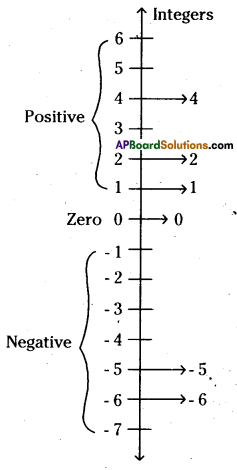Question 2.
Represent opposite integers of – 200 and + 400 on integer number line.
Solution:
∴ Opposite integer (additive inverse) of – 200 is 200.
Opposite integer (additive inverse) of +400 is – 400.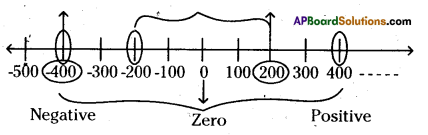Let’s Think (Page No. 50)

Question 1.
For any two integers, say 3 and 4, we know that 3 < 4.
Is it true to say -3 < -4? Give reason.
Solution:
On the number line, the value of a number increases as we move to right and decreases as we move to the left. As -3 lies right to -4 on the number line.
So, -3 < -4 is not true.(Pg. No. 52)

Question 1.
What is additive inverse of 7 ?
Solution:
Additive inverse of 7 is -7.

Question 2.
What is additive inverse of -8 ?
Solution:
Additive inverse of -8 is 8.

Let’s Explore (Page.No. 52)

Question 1.
Find the value of the following using a number line.
i) (-3) + 5 ii) (-5) + 3
Make two questions on your own and solve them using the number line.
Solution:
i) (-3) + 5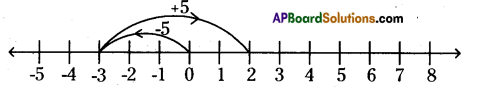On the number line, we first move 3 steps to the left of 0 to reach -3.
Then, we move 5 steps to the right of -3 and reach +2. So, (-3) + 5 = 2

ii) (-5) + 3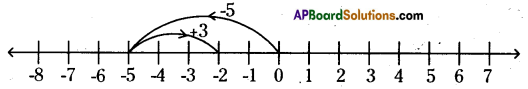On the number line, we first move 5 steps to the left of 0 to reach -5. Then, we move 3 steps to the right of -5 and reach -2. So, (-5) + 3 = – 2

iii)(+6) + (-3)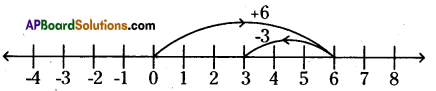On the number line, we first move 6 steps to the right of 0 to reach +6.
Then, we move 3 steps to the left of 6 and reach +3. So, (+6) + (-3) = 3

iv) (-4) + (-3)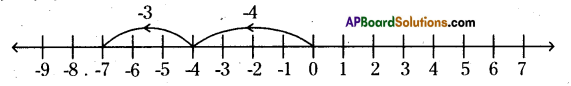On the number line, we first move 4 steps to the left of 0 to reach -4. Then, we move 3 steps to the left of -4 and reach -7.
So (-4) + (-3) = -7.

Question 2.
Find the solution of the following:
i) (+5) + (-5) (ii) (+6) + (-7) (iii) (-8) + (+2)
Solution:
i) (+5) + (-5) = 0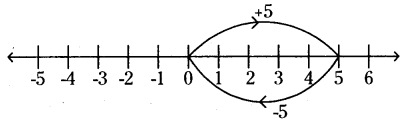On the number line, we first move 5 steps to the right of 0 to reach +5.
Then, we move 5 steps to the left of +5 and reach 0.ii) (+6) + (-7) = -1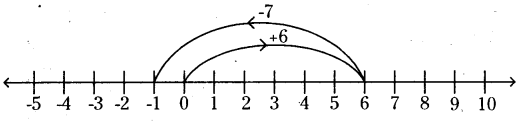On the number line, we first move 6 steps to the right of 0 to reach +6.
Then, we move 7 steps to the left of +6 and reach -1.

(iii) (-8) + (+2)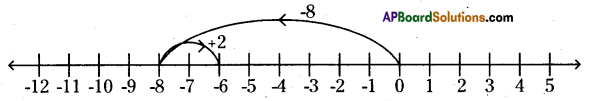On the number line, we first move 8 steps to the left of 0 to reach -8. Then, we move 2 steps to the right of -8 and reach -6.

iv)(-4) + (+8) = +4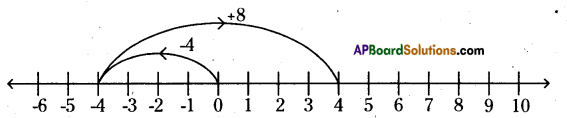On the number line, we first move 4 steps to the left of 0 to reach -4. Then, we move 8 steps to the right of -4 and reach +4.

v) (+3) + (-4) = -1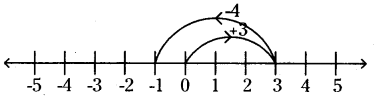On the number line, we first move 3 steps to the right of 0 to reach +3. Then, we move 4 steps to the left of +3 and reach -1.

vi) (+5) + (-6) = -1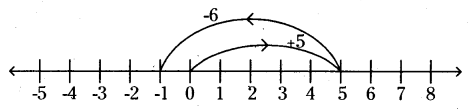On the number line, we first move 5 steps to the right of 0 to reach +5. Then, we move 6 steps to the left of +5 and reach -1.vii) (+4) + (-4) = 0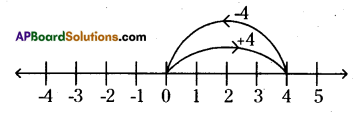On the number line, we first move 4 steps to the right of 0 to reach +4. Then, we move 4 steps to the left of +4 and reach 0.

viii) (-6) +(+4) =-2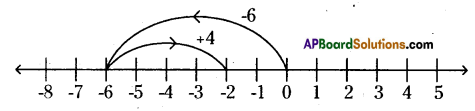On the number line, we first move 6 steps to the left of 0 to reach -6. Then, we move 4 steps to the right of -6 and reach -2.
So, (-6) + (+4) = -2

Let’s Explore (Page No. 55)

Question 1.
Take any two integers a and b. Check whether a+b is also an integer.
Case (i) : Consider two integers 3 and -2 (Positive and negative)
Sum = 3 + (-2) = +1 + 2 – 2 = +1 + 0 = +1 is also an integer.
Case (ii) : Consider two ihtegers 5 and 6 (Both are positive)
Sum = 5 +6 = + 11 is also an integer
Case (iii) : Consider two integers -4 and -6 (Both are negative)
Sum = -4 + (-6) = -4 -6 = -10 is also an integer
Case (iv) : Consider two integers -5 and 4 (Negative and positive)
Sum = -5 + 4 = -1 -4 + 4 = -1 + 0 = -1 is also an integer.
So, if a and b are integers, then their sum a + b is also an integer. Integers are closed under addition.

Question 2.
Check the following Properties on integers, a, b, c are any integers.
i) Closure Property under subtraction ‘
ii) Commutative Property under addition and subtraction (a + b = b + a ?, a – b = b – a?)
iii) Associative Property under addition and subtractioji.
(a + b) + c = a + (b + c) ? (a – b).- c = a – (b – c)?
Solution:
i) Closure Property under subtraction :
Case (i) : Consider two integers 4, -5 (positive and negative)
Then, difference a – b = 4 – (-5) = 4 + 5 = + 9is also an integer.
Case (ii) : Consider two integers 3, 8 (Both are positive)
Then, difference a – b = 3 – (+8) = 3-8
= +3 – 3 – 5 = 0 – 5 = -5is also an integer.
Case (iii) : Consider two integers -2, -6 (Both are negative) .
Then, difference a – b = -2 – (-6) = -2 + 6
= -2 + 2 + 4 = 0 + 4 = +4 is also an integer.

Case (iv) : Consider two integers -3, 2 (Negative and positive)
Then, difference a – b = -3 – (+2) = -3 -2 = -5 is also an integer.
So, if a and b are any two integers, then their difference a – b is also an integer. Integers are closed under subtraction.ii) Commutative Property under addition and subtraction :
(a + b = b + a, a-b = b-a)
(A) Case (Q : Consider two integers-3 and 5 (Negative and positive)
Then, a + b = -3 + (+5)
= -3 + 5 = – 3 + 3 + 2 = 0 + 2 = + 2
b + a = +5 + (-3) = +2 + 3- 3 = +2 + 0 = + 2
∴ a + b = b + a

Case (ii) : Consider two integers +4 and +2 (Both are positive)
Then, a + b = +4 + (+2) = +4 + 2 = + 6 ‘b + a = +2 + (+4) = +2 + 4 = + 6
∴ a + b = b + a

Case (iii) Consider two integers -5 and -3 (Both are negative) Then, a + b = -5 + (-3) = -5 – 3 = -8 b + a = -3 + (-5) = – 3 – 5 = -8
∴ a + b = b + a

Case (iv) : Consider two integers +4 and -1 (Positive and negative)
Then, a + b = +4 + (*1) = +4 -1 = +3 + 1 -1 = +3 + 0 = +3 b + a = -1 + (+4) =-l + 4 = -l + l+ 3 = 0 + 3 = + 3
∴ a + b = b + a
So, integers are commutative under addition.

(B) Consider two integers -4 and +6 (Negative and positive)
Then, a – b = -4 – (+6) = -4 – 6 = -10 b-a = + 6-(-4) = +6 +4 = +10 -10*10
a – b ≠ b – a
So, integers are not commutative under subtraction.

iiO Associative property under addition and subtraction :
(a + b) + c = a + (b + c) ; (a – b) – c = a – (b – c)
(A) Case (i) : Consider any three integers 2, 4, -5
(a + b) + c = (2 + 4) + (-5) = 6 – 5 = +1 + 5- 5 = +1 + 0 = +1
a + (b + c) = 2 + (4 + (-5)) = 2 + (4 – 5) = 2 + (4 – 4 -1)
= 2 + (0-1) = + 2 – 1 = + 1 + 1 – 1 = +1 + 0 = +1
∴ (a + b) + c = a + (b + c)

Case (ii) : Consider any three integers +2, -5, +3
Then, (a + b) + c = [2 + (-5)] + 3 = [+2 -5] + 3 = +2 -2 -3 + 3 = 0 + 0 = 0
a + (b + c) = +2 + [( – 5)+3] = +2 + [-2 – 3 + 3] = +2 + (-2 + 0) = +2 -2 = 0
∴ (a + b) + c = a + (b + c)

Case (iii) : Consider any three integers 3, 4, 6
Then, (a + b) + c = [2 + (-5)] + 3 [+2-5] + 3
a + (b + c) = 3 + (4 + 6) = 3 + 10 = + 13
∴ (a + b) + c = a + (b + c)Case (iv) : Consider any three integers -4, -2, +5
Then, (a + b) + c = [-4 + (-2)] + 5 = [-4 -2] + 5 = -6 + 5
= -1-5 + 5 = – 1 + 0 = – 1
a + (b + c) = -4 + [(-2) + 5] = -4 + [-2 + 5]
= _4 + [_2 + 2 +3] = -4 + 0 + 3 =-1-3+ 3
= -1 + 0 = -1
∴ (a + b) + c = a + (b + c)

Case (v) : Consider any three integers-3, 4, 1
Then, (a + b) + c = (-3 + 4) + 1 = (-3 + 3 + 1) + 1 = 0 + 1 + 1 = + 2
a + (b + c) = -3 + (4 + 1) = -3 + 5 = -3 + 3 + 2 = 0 + 2 = +2
∴ (a + b) + c = a + (b + c)

Case (vi) : Consider any three integers -2, 6, -7
Then, (a + b) + c = (-2 + 6) + (-7) = (-2 + 2 + 4) + (-7) = 0 + 4 – 7
= + 4 – 4 – 3 = 0 – 3 = -3
a + (b + c) = -2 + [6 + (-7)] = -2 + (6 – 7) = -2 + [+6 – 6 -1]
= -2 + (-1) = -2 -1 = -3
∴ (a + b) + c = a + (b + c)

Case (vii) : Consider any three integers +6, -3, -1
Then, (a + b) +c = [+6 + (-3)] + (-1) = (6 – 3) – 1 = (+3 +3 -3) -1
=+3-1 =+2 + 1 – 1 =+2 + 0 = + 2
a + (b + c) = +6 + [-3 + (-1)] = 6 + [-3 – 1] = 6 + (-4) .
= +2 + 4 – 4 = 2 + 0 = + 2
∴ (a + b) + c = a + (b + c)

Case(viii)
Consider any three integers -4, -1, -7
Then, (a + b) + c = [-4 + (-1)] + (-7) = (-4 -1) – 7 = -5 -7 = -12
a + (b + c) = -4 + [(-1) + (-7)] = -4 + [-1 -7] = -4 + (-8)
= -4 – 8 = -12
∴ (a + b) + c = a + (b + c)
From all the above cases we conclude that, integers are associative under addition.(B) Consider any three integers +5, -4, 1
Then, (a – b) – c = (+5 – (-4)) – (+1) = (5 + 4) – 1 .
= + 9 – 1 = + 8 + 1 – 1 = + 8 + 0 = + 8
a – (b – c) = +5 – [- 4 – (+1)] = + 5 – [-4 – 1]
= + 5 – [ -5] =+ 5 + 5 = + 10
+ 8 ≠ +10
∴ (a – b) – c ≠ a – (b – c)
So, integers are not associative under subtraction.